UPSC  >  Important Concepts: Number System - 1

# Important Concepts: Number System - 1 Notes | Study CSAT Preparation for UPSC CSE - UPSC

## Document Description: Important Concepts: Number System - 1 for UPSC 2022 is part of CSAT Preparation for UPSC CSE preparation. The notes and questions for Important Concepts: Number System - 1 have been prepared according to the UPSC exam syllabus. Information about Important Concepts: Number System - 1 covers topics like Introduction, Face Value and Place Value, Types of Numbers and Important Concepts: Number System - 1 Example, for UPSC 2022 Exam. Find important definitions, questions, notes, meanings, examples, exercises and tests below for Important Concepts: Number System - 1.

Introduction of Important Concepts: Number System - 1 in English is available as part of our CSAT Preparation for UPSC CSE for UPSC & Important Concepts: Number System - 1 in Hindi for CSAT Preparation for UPSC CSE course. Download more important topics related with notes, lectures and mock test series for UPSC Exam by signing up for free. UPSC: Important Concepts: Number System - 1 Notes | Study CSAT Preparation for UPSC CSE - UPSC
 Table of contents Introduction Face Value and Place Value Types of Numbers
 1 Crore+ students have signed up on EduRev. Have you?

Introduction

• A system is a set of principles or procedures according to which something is done.
• Number System is a set of principles according to which numbers are represented and various arithmetic operations like addition, subtraction, multiplication, etc. are applied to them. This lays down the basic rules on how various digits and symbols are used.
• Whenever we change the principles, we get a new set of the number system. This results in various types of number systems.
• Some of the examples of the number systems are: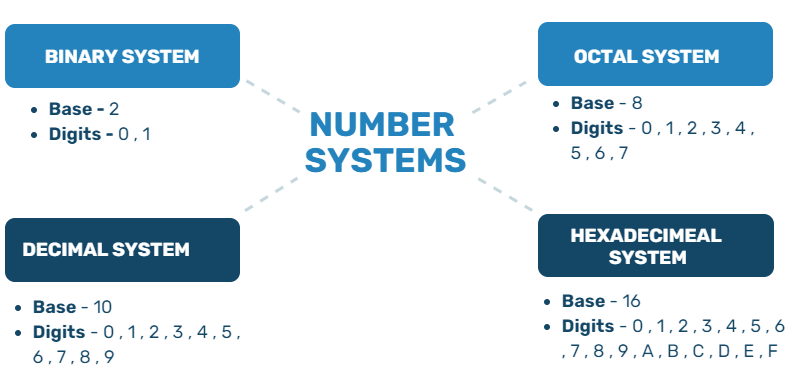Types of Number Systems(i) Base is defined as the number of digits we use to represent the numbers.
(ii) Digits are the tools that are used to represent numbers.

We will be talking about various number systems and the bases in subsequent chapters. The standard number system that we use across the world is the decimal number system. Decimal Number System has a base "10" as it uses 10 digits (0, 1, 2, 3, 4, 5, 6, 7, 8, 9) to represent all the numbers.

Face Value and Place Value

• Face Value: The Value of the digit itself is referred to as the face value of a particular Digit. In the decimal number system, we use the 10 digits, as mentioned above. Wherever used, the face value remains the same.
Example: The face value of 5 is 5 in 568 and 1285 and even 58738.
• Place: The place value of a number depends on the place it is at. The various places and the corresponding multipliers of the places in a decimal number system are listed below. We will be starting from leftmost and move the right place by place.
Example: For a number 786543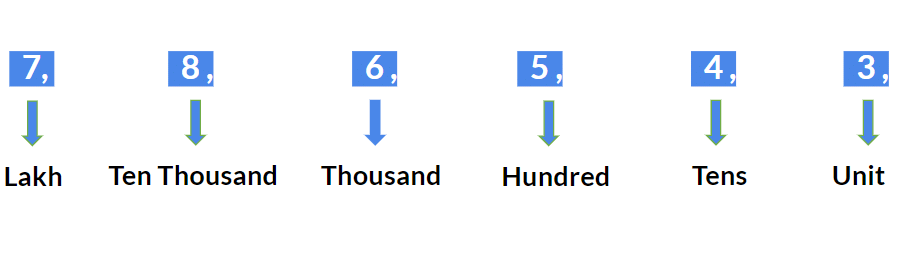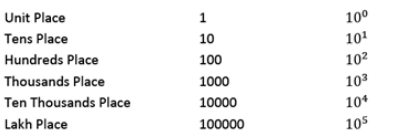• Place Value of a digit in a particular number system is defined as the face value of the number multiplied by the corresponding place. In the decimal system, the value of the place will be as mentioned in the table above.
Example: The place value of 5 is 500 in 568 and 5 in 1285 and 50000 in 58738.
Question for Important Concepts: Number System - 1
Try yourself:What is the difference in the two Place Values and Two Face Values of digit 7 in the given number - 78687

In this chapter we are going to talk about the Numbers, their properties and their classifications. As discussed earlier all these properties will be limited to decimal number system only since that is the basis of all the question asked. The concepts discussed in this chapter will be your first step towards a general understanding of the mathematics requirements to clear any entrance exams. As we proceed you will realize that you have already learnt most of these concept in school.

Types of Numbers

1. Natural Number

The primary application of mathematics is counting. That is where the very first category of numbers comes in, i.e. natural numbers or commonly as known as counting Numbers.

Features of Natural Numbers

• These are the complete numbers, i.e. fractions/decimals, are not included.
• Zero is not included.
• Negatives are not included.
• They are represented by "N."
• N = {1, 2, 3, 4, 5.........infinity}

Question for Important Concepts: Number System - 1
Try yourself:Choose the set of Numbers which contains only Natural Numbers

2. Whole Numbers

Natural numbers along with 0, form a set of numbers known as Whole numbers. Since '0' is a representation of the absence of anything and is not used for counting, thus they are not called the counting numbers.

Features of Whole Numbers

• These are the complete numbers, i.e. fractions/decimals, are not included.
• Zero is included.
• Negatives are not included.
• They are represented by "W".
• W = {0, 1, 2, 3, 4, 5.........infinity}.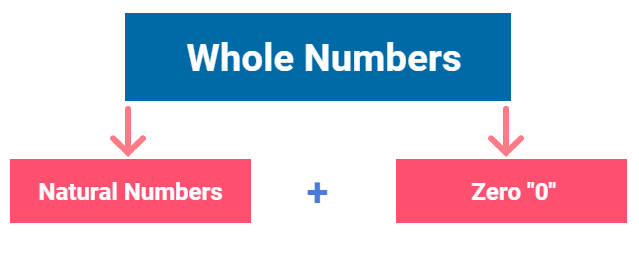Question for Important Concepts: Number System - 1
Try yourself:Choose the set of Numbers which contains only Whole Numbers

3. Integers

Numbers less than 'zero' are represented with a negative sign along with them and are referred to as negative numbers.
Whole numbers, along with negative of natural numbers form a set of numbers known as integers.
Features of Integers

• These are the complete numbers, i.e. fractions/decimals, are not included.
• Zero is included.
• Negatives are included.
• They are represented by "I".
• I = {- infinity.......-3, -2, -1, 0, 1, 2, 3 ......... infinity}.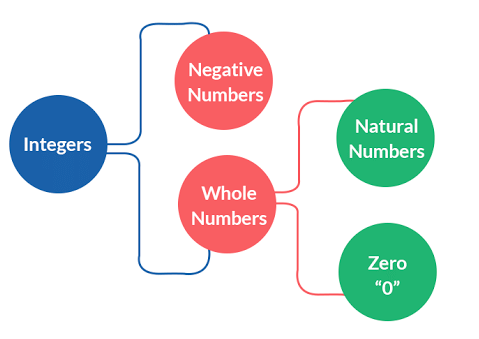Question for Important Concepts: Number System - 1
Try yourself:Choose the set of Numbers which contains only Integers

4. Fractions

The word ‘fraction’ has been derived from the Latin ‘fractus’ which means “broken”. Fraction represent part of a whole. When we divide a whole into pieces, each part is a fraction of the whole.
Example: 1/2, 3/5, 2/7, 10/21

A fraction has two parts. The number on the top of the line is called the numerator. The number below the line is called the denominator.

Types of Fractions

Fractions are generally of two types on the basis of representation:
(i) Simple Fraction
Example: 30/7
(ii) Mixed Fraction
Example: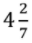Question for Important Concepts: Number System - 1
Try yourself: Convert the mixed fraction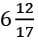into a simple fraction.

Question for Important Concepts: Number System - 1
Try yourself:Convert the simple fraction 112/19 into a mixed fraction.

5. Decimal Numbers

These are nothing but a form of representation of fractions. We make the value of the denominator as unity, i.e. "1". They can be both negative and positive.
Example: 2.5, 1.25, 1.3333 ......., 2.666....., 2.8284.....

Types of Decimal Numbers

Decimal numbers are majorly divided into two categories:

• Terminating Decimal Numbers - The decimal numbers with a definite end.
Example: 2.5, 1.25
• Non-Terminating Decimal Numbers - The decimal numbers without a definite end.
Example: 1.3333 ......., 2.666....., 2.8284.....
Non-Terminal Decimal Numbers are further divided into two categories:
(i) Recurring non-terminating decimal numbers: The non-terminating decimal numbers with digits after the decimal repeating again and again.
(ii) Non-recurring non-terminating decimal numbers: The non-terminating decimal numbers with digits after the decimal do not repeat but have a completely random pattern. These are the irrational numbers which we will discuss ahead in the same chapter.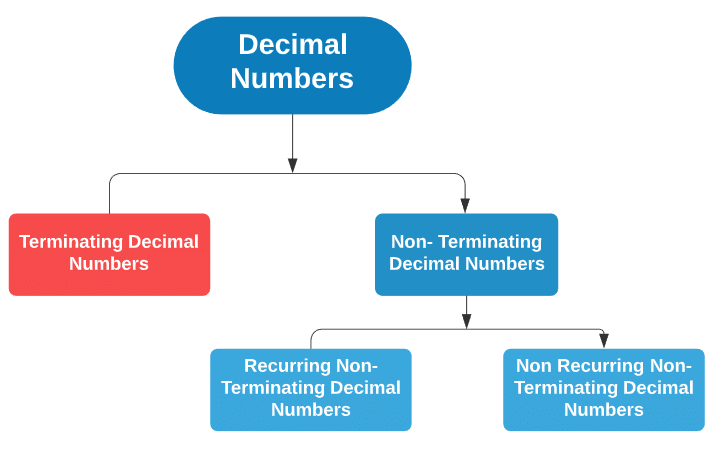Classification of Decimal Numbers

6. Rational Numbers

All those numbers which can be represented in the form of p/q where p and q are integers with q ≠ 0 are known as Rational numbers. All the integers, fractions, terminating decimal numbers and non-terminating recurring decimal numbers fall under the category of Rational Numbers.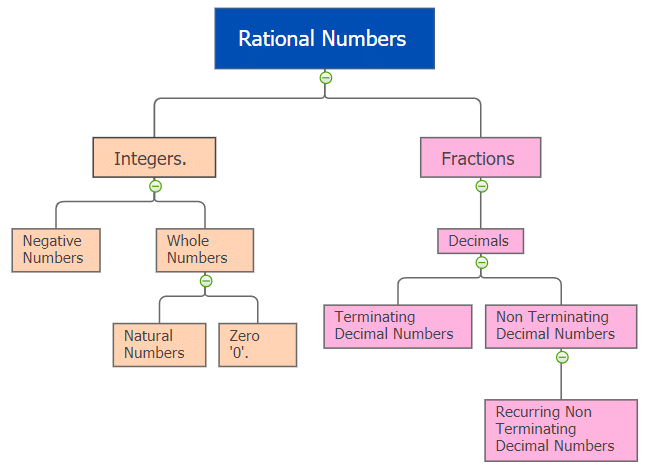Classification of Rational Numbers

7. Irrational Numbers

All those numbers which are not rational are known as irrational numbers. Non-reciprocating non-terminating decimal numbers are irrational numbers. Square Roots, Cube Roots, etc. of natural numbers turn out to be irrational numbers.
Example: √3 = 1.7320.... , √8 = 2.8284 ......, 3√4 = 1.5874 .......

Converting a Terminating Decimal Number in p/form:

Example: Convert 80.125 in p/q form

• Count how many digits are thereafter decimal, 3 in this case.
• Remove the decimal and divide the number so received by as many 10's as the counting in step 1.
80.125 = 80125/1000 = 641/8

Question for Important Concepts: Number System - 1
Try yourself:Convert 128.8888 in p/q form

Converting a non-terminating recurring decimal number in p/q form:

Example: Convert 80.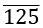in p/q form.

• Let the number be equal to x
x = 80.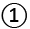• Count how many digits are thereafter decimal, 3 in this case.
• Multiply by as many tens on both sides, i.e. 1000, in this case.
1000x = 80125.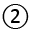• Subtractfrom999x = 80045
x = 80045 / 999

Example: Convert 80. 1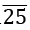in p/q form.

• Let  80.1= x• Multiply both sides by 100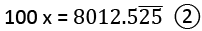• Subtractfrom99x = 7922.4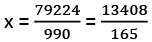Question for Important Concepts: Number System - 1
Try yourself:Convert 16.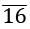in p/form

Question for Important Concepts: Number System - 1
Try yourself:Convert 23.0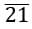in p/q form

The process we have applied in the examples above is too time-consuming. We need a method that can do the same process in much less time. For that, we have a formula with which you can have the answer in a single step.

Every terminating recurring decimal number has 3 parts:
(Taking the example of: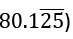(i) Digits before the decimal (80).
(ii) No. of digits with the bar on them after the decimal (2).
(iii) No. of digits without a bar on them after the decimal (1).

Rational form: All the digits without decimal written once - All the digits without bar written once / as many 9's as no. of digits with bar on them after decimal followed by as many 0's no. of digits without bar on them after decimal.

Example: Convert 80.in p/q form

• All the digits are written once = 80125
• All the digits without bar written once = 80
• No. of digits with bar after decimal = 3
• No. of digits without bar after decimal = 0
• Rational form = (80125 - 80) / 999 = 80045 / 999

Example: Convert 80.1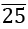in p/q from

• All the digits are written once = 80125
• All the digits without Bar written once = 801
• No. of digits with bar after decimal = 2
• No. of digits without bar after decimal = 1
• Rational form = (80125 - 801) / 990 = 79224 / 990

Question for Important Concepts: Number System - 1
Try yourself:Convert 16.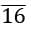in p/q from

Question for Important Concepts: Number System - 1
Try yourself: Convert 23.0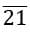in p/q form

8. Real Numbers

• Rational numbers, as well as irrational numbers together, form the real numbers. As per the definition, all the numbers which can be represented on a number line are known as real numbers.
• All the categories which we have discussed till now all fall in the set of Real numbers, including the irrational numbers. They can also be represented on a number lines.

Question for Important Concepts: Number System - 1
Try yourself:Choose the set of Numbers which doesn't contains only Real Numbers

9. Imaginary Numbers

• All those numbers that actually do not exist in a number system but assume their presence for the sake of calculations are known as imaginary numbers.
• They cannot be represented on number lines. They are also called complex numbers.
Example: √−3, √−16, 4√−8

Note: The study of complex numbers is not important from Aptitude Testing point of view. So, we will not take this topic any further.

The document Important Concepts: Number System - 1 Notes | Study CSAT Preparation for UPSC CSE - UPSC is a part of the UPSC Course CSAT Preparation for UPSC CSE.
All you need of UPSC at this link: UPSC

## CSAT Preparation for UPSC CSE

72 videos|64 docs|92 tests
 Use Code STAYHOME200 and get INR 200 additional OFF

## CSAT Preparation for UPSC CSE

72 videos|64 docs|92 tests

### How to Prepare for UPSC

Read our guide to prepare for UPSC which is created by Toppers & the best Teachers

Track your progress, build streaks, highlight & save important lessons and more!

,

,

,

,

,

,

,

,

,

,

,

,

,

,

,

,

,

,

,

,

,

;